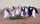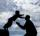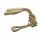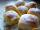# Benches

The park has 64 benches. Occupied are by 18 more than empty. How many benches are occupied and empty ?

Result

o =  41
p =  23

#### Solution:

o+p=64
o=18+p

o+p = 64
o-p = 18

o = 41
p = 23

Calculated by our linear equations calculator.Our examples were largely sent or created by pupils and students themselves. Therefore, we would be pleased if you could send us any errors you found, spelling mistakes, or rephasing the example. Thank you!

Leave us a comment of this math problem and its solution (i.e. if it is still somewhat unclear...):Be the first to comment!#### Following knowledge from mathematics are needed to solve this word math problem:

Do you have a linear equation or system of equations and looking for its solution? Or do you have quadratic equation?

## Next similar math problems:

1. Apples 3Julka has 5 apples more than Hugo and four apples less than Annie. Hugo has 17 apples. How many apples has Julka and how Annie?
2. Rabbit familyRabbit family ate 32 pieces of carrots, small one ate 6 pieces, dad 5 more than the mother. How much ate mom?
3. Temperature 2Sunday's high temperature was 3 degrees higher than Saturday's. On Monday, the temperature fell 5 degrees, then rose 7 degrees on Tuesday and 4 more on Wednesday. Then it fell 17 degrees to a record low of 31 on Thursday. What was the temperature on SaturdThe fruit basket is five times heavier than the empty basket. Filled is 20kg heavier than empty. Determine the weight of the fruit in the basket.
5. Father and sonFather is 44 years old, his son 16 years. Determine how many years ago was the father five times older than the son.
6. Forest nurseryIn the forest nursery after winter, they found that 1/10 stems died out of them. For them, they land 193 new spruces. How many spruces are in the forest nursery?
7. ToysThe toy shop sold the same number of balls for 62 CZK and skipping ropes for 34 CZK. They income was 576 CZK. How many sold balls and how many skipping ropes?
8. Jam cakesMom baked a third of plum jam cakes, one third cheesecakes and 18 poppy. How many cakes she had bake?
9. PopsiclesFrancis went to buy ice lollies. If he buy 8 popsicles he missed 4 USD. When he buy 7 popsicles, got back 1 USD. How many USD was a popsicle?
10. Eq1Solve equation: 4(a-3)=3(2a-5)
11. Six te 2If 3t-7=5t, then 6t=
12. Simple equation 1035= 7*3*x what is x?
13. If-then equationIf 5x - 17 = -x + 7, then x =
14. Simple equation 8Solve the following equation: 36=-(1+7x)-6(-7-x)
15. NumberDetermine unknown number if you know that difference between five times and triple of number is 42.
16. Jan and DanJan and Dan had the same money. Jan bought 5 workbooks and left him 15 CZK. Dan 6 and left him nothing. How much money have in total?Added together and write as decimal number: LXVII + MLXIV